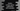# Python numpy square method explanation with examples## Python numpy square method explanation with examples:

Python numpy square method is used to find the squares of each element in an array. It takes an array_like input and returns an newly created array.

In this post, we will learn how to use the numpy.square method with different types of array inputs.

### Definition of numpy square method:

This method is defined as like below:

``numpy.square(x, /, out=None, *, where=True, casting='same_kind', order='K', dtype=None, subok=True[, signature, extobj]) = <ufunc 'square'>``

Where,

• x is the input array_like data.
• out is an optional value. It can be ndarray, None or tuple of ndarray and None. It should have the same shape as the input data. This is a location to put the result. If this is not provided, a new array is created and returned.
• where is another optional value. This is a condition that is broadcast over the elements of the input array.

It returns a new array or array_like object with each element as the square. It has the same shape and dtype as the input array.

### Example of numpy square method with an integer array:

Let’s try it with an integer array:

``````import numpy as np

given_arr = [1, 2, 3, 4, 5, 6, 7]

print(np.square(given_arr))``````

If you run this program, it will print:

``[ 1  4  9 16 25 36 49]``

So, as you can see here, all elements are square of the elements.

We can also use it with any other dimension arrays. For example, let’s try it with a 2-D array:

``````import numpy as np

given_arr = [[1, 2, 3], [4, 5, 6], [7, 8, 9]]

print(np.square(given_arr))``````

It will print:

``````[[ 1  4  9]
[16 25 36]
[49 64 81]]``````

### Example with complex numbers array:

Similar to the above example, we can also use an array with complex numbers. For example:

``````import numpy as np

given_arr = [1 + 2j, 3 - 4j]

print(np.square(given_arr))``````

It will print:

``[-3. +4.j -7.-24.j]``